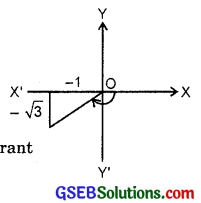# GSEB Solutions Class 11 Maths Chapter 5 Complex Numbers and Quadratic Equations Ex 5.2

Gujarat Board GSEB Textbook Solutions Class 11 Maths Chapter 5 Complex Numbers and Quadratic Equations Ex 5.2 Textbook Questions and Answers.

## Gujarat Board Textbook Solutions Class 11 Maths Chapter 5 Complex Numbers and Quadratic Equations Ex 5.2

Find the modulus and the argument of each of the complex numbers in questions 1 to 2:
1. z = – 1 – i$$\sqrt{3}$$
2. z = – $$\sqrt{3}$$ + i
Solutions to questions 1 to 2:
1. z = – 1 – i$$\sqrt{3}$$
∴ Let – 1 – i$$\sqrt{3}$$ = r(cos θ + isinθ)
∴ – 1 = rcos θ and $$\sqrt{-3}$$ = r sin θ
4 = 1 + 3 = r2(cos2 θ + sin2 θ) = r2
∴ r = $$\sqrt{4}$$ = 2
tan θ = $$\frac{\sqrt{3}}{-1}$$ = – $$\sqrt{3}$$.
where θ lies in third quadrant.
∴ θ = – 180° + 60° = – 120° = – $$\frac{2π}{3}$$
|z| = 2, arg z = – $$\frac{2π}{3}$$

2. z = – $$\sqrt{3}$$ + i = r(cos θ + 180°)
∴ rcos θ = – $$\sqrt{3}$$, r sin θ = 1
Squaring and adding ($$\sqrt{3}$$)2 + 1 = 4
∴ r = 2, we gettan θ = $$\frac{-1}{\sqrt{3}}$$ ⇒ θ lies in II Quadrant
∴ θ = 180° – 30° = 150° = $$\frac{5π}{6}$$
∴ |z| = 2, arg z = $$\frac{5π}{6}$$.Convert each of the complex numbers given in questions 3 to 8 in the polar form:
3. 1 – i
4. – 1 + i
5. – 1 – i
6. – 3
7. $$\sqrt{3}$$ + i
8. i
Solutions to questions 3 to 8:
3. If 1 – i = r(cos θ + isin θ)
∴ rcos θ = 1, rsin θ = – 1
12 + 12 = r2
∴ r = $$\sqrt{2}$$
and tan θ = $$\frac{- 1}{1}$$ = – 1.
∴ θ lies in IV quadrant, since sin θ is negative and cos θ is positive.
∴ θ = – 45° = – $$\frac{π}{4}$$.
∴ Polar form of 1 – i is
$$\sqrt{2}$$[cos (- $$\frac{π}{4}$$) + isin (- $$\frac{π}{4}$$)]4. z = – 1 + i = r(cos θ + isin θ)
∴ rcos θ = – 1, r sin θ = 1
r2 = (- 1)2 + 12 = 2 is r = $$\sqrt{2}$$.
Here, sin θ is +ve and cos θ is – ve. Therefore, θ lies in the second quadrant.
i.e; θ = π – $$\frac{π}{4}$$ = $$\frac{3π}{4}$$.
∴ z = $$\sqrt{2}$$(cos $$\frac{3π}{4}$$ + isin $$\frac{3π}{4}$$).

5. z = – 3 = r(cos θ + i sin θ)
∴ rcos θ = – 1, r sin θ = – 1
r2 = (- 1)2 + (- 1)2 = z
∴ r = $$\sqrt{2}$$.

6. z = – 3 = r(cos θ + i sin θ)
∴ rcos θ = – 3, rsin θ = 0.
Squaring and adding, we get r2 = (- 3)2
∴ r = 3.
tan θ = 0 ⇒ θ = π [∵ cos π = 0]
∴ – 3 = 3(cos π + isin π).7. r = $$\sqrt{3}$$ + i = r(cos π + isinπ)
∴ rcos θ = $$\sqrt{3}$$, r sin θ = 1
Also, tan θ = $$\frac{1}{\sqrt{3}}$$. Also, sin θ and cos θ both are positive.
∴ θ = 30° = $$\frac{π}{6}$$.
Polar form of z is 2(cos $$\frac{π}{6}$$ + isin $$\frac{π}{6}$$).
Now, sin θ = 1, cos θ = 0 at θ = $$\frac{π}{2}$$.
∴ Polar form of z is cos $$\frac{π}{2}$$ + isin $$\frac{π}{2}$$.